# Electronics and Communication Engineering - Automatic Control Systems

### Exercise :: Automatic Control Systems - Section 2

31.

For quadratic factor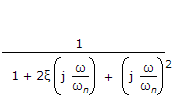. In Bode log magnitude plot the high frequency asymptote is a straight line having the slope

Explanation:

For quadratic factor in denominator, slope at high frequencies is - 40 dB/decade.

32.

For op-amp circuit of the given figure, Eo(s)/Ei(s) =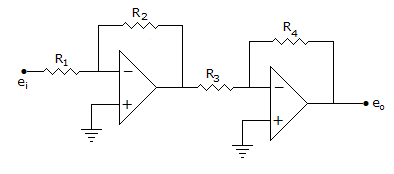A.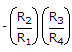B.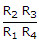C.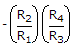D.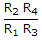Explanation: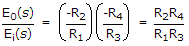.

33.

Assertion (A): When the phase margin of a control system is improved using a lead network, noise transmission increases.

Reason (R): Phase lead compensation increases bandwidth.

 A. Both A and R are correct and R is correct explanation of A B. Both A and R are correct but R is not correct explanation of A C. A is correct but R is wrong D. R is correct but A is wrong

Explanation:

More bandwidth means more noise.

34.

In liquid flow system the capacitance of a water tank is defined as

 A.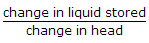B.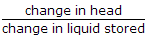C.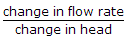D. none of the above

Explanation:

Electrical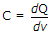and dQ is analogous to change in amount of liquid and dv is analogous to change in head.

35.

For a first order system having transfer function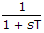, the unit ramp response is

 A. T(1 - e-t/T) B. t - T + T e-t/T C. t + T + T e-t/T D. t + 2T e-t/T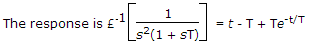.